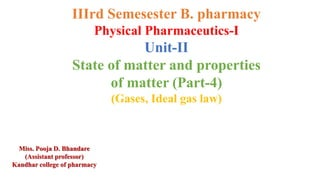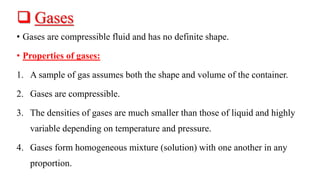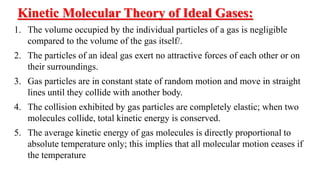Diese Präsentation wurde erfolgreich gemeldet.

# State of matter and properties of matter (Part-4) (Gases, Ideal gas law)

Anzeige
Anzeige
Anzeige
Anzeige
Anzeige
Anzeige
Anzeige
Anzeige
Anzeige
Anzeige
Anzeige×

1 von 14 Anzeige

# State of matter and properties of matter (Part-4) (Gases, Ideal gas law)

Gases, Properties of gases, Kinetic Molecular Theory of Ideal Gases, The Gas laws:1.Boyle’s Law ( The Pressure – Volume relationship), 2.Charles’s law( The Temperature- Volume relationship), 3. Gay- Lussac’s law( The Pressure- Temperature relationship), 4. Avogadro’s Law ( The Volume – Amount relationship), Ideal Gas Law:

Gases, Properties of gases, Kinetic Molecular Theory of Ideal Gases, The Gas laws:1.Boyle’s Law ( The Pressure – Volume relationship), 2.Charles’s law( The Temperature- Volume relationship), 3. Gay- Lussac’s law( The Pressure- Temperature relationship), 4. Avogadro’s Law ( The Volume – Amount relationship), Ideal Gas Law:

Anzeige
Anzeige

### State of matter and properties of matter (Part-4) (Gases, Ideal gas law)

1. 1. IIIrd Semesester B. pharmacy Physical Pharmaceutics-I Unit-II State of matter and properties of matter (Part-4) (Gases, Ideal gas law) Miss. Pooja D. Bhandare (Assistant professor) Kandhar college of pharmacy
2. 2.  Gases • Gases are compressible fluid and has no definite shape. • Properties of gases: 1. A sample of gas assumes both the shape and volume of the container. 2. Gases are compressible. 3. The densities of gases are much smaller than those of liquid and highly variable depending on temperature and pressure. 4. Gases form homogeneous mixture (solution) with one another in any proportion.
3. 3. Kinetic Molecular Theory of Ideal Gases: 1. The volume occupied by the individual particles of a gas is negligible compared to the volume of the gas itself/. 2. The particles of an ideal gas exert no attractive forces of each other or on their surroundings. 3. Gas particles are in constant state of random motion and move in straight lines until they collide with another body. 4. The collision exhibited by gas particles are completely elastic; when two molecules collide, total kinetic energy is conserved. 5. The average kinetic energy of gas molecules is directly proportional to absolute temperature only; this implies that all molecular motion ceases if the temperature
4. 4. The Gas laws: 1. Boyle’s Law ( The Pressure – Volume relationship) : This law states that the volume of a fixed amount of gas at a constant temperature is inversely proportional to the gas pressure. The pressure volume relationship. Robert Boyle, in 1662 V α 1/P V = k/P For a given sample of gas under two different sets of condition at constant P1 V1 = P2 V2
5. 5. 2. Charles’s law( The Temperature- Volume relationship) • Charles in 1787 investigated that the gas such as hydrogen, carbon and oxygen expand to an equal amount upon heating from 0°C to 80°C at constant pressure .However, Gay-Lussac in 1802 showed that volume of all gases increases with each 1°C was approximately equal to 1/273.15 volume of gas at 0°C
6. 6. • Charles’s law state that the pressure of a fixed amount of gas at a constant at a fixed pressure, the volume of gas is proportional to temperature of gas. Vα T V = kT For a given sample of gas under two different sets of condition at constant V1 /T1 = V2 / T2 = K • At any given pressure, the plot of volume vs temperature. Yields a straight line. • The temperature increases, the gas expand ( volume increases ) • The temperature decreases, the gas expand ( volume decreases)
7. 7. 3. Gay- Lussac’s law( The Pressure- Temperature relationship • This law is a special case of ideal gas law. This law applies to ideal gases held at a constant volume allowing only the pressure and temperature to change. P1 / T1 = P2 / T2
8. 8. 3. Avogadro’s Law ( The Volume – Amount relationship • Amedeo Avogadro- complemented the studies of Bolyle, Charles and Gay- Lussac. He published a hypothesis that started: • At the same temperature and pressure, equal volume of different gases contain the same number of molecules. • The Volume of any given gas must be proportional to the number of molecules present
9. 9. • Avogadro law : this law states that the volume of a sample gas is directly proportional the number of moles in the sample at constant temperature and pressure. Vα n Or, V1 / n1 = V2 / n2
10. 10. 1. Boyles Law = Vα 1 𝑃 ( constant temperature) 2. Charles’s Law = Vα T ( constant pressure ) 3. Avogadro’s Law = Vα n ( constant temperature and pressure) GAS LAW
11. 11. Ideal Gas Law: • The ideal gas law relates to the volume and pressure of a gas at constant temperature. On combining Boyle’s law, Charles’s law, Gay- Lussac law and Avogadro’s law we find that the volume of gas depends on pressure, temperature and number of moles of gas in the container. • Summary: Boyle’s Law : V α 1 𝑃 ( when n and T are held constant) Charles’s Law: V α T (when n and P are held constant) Avogadro’s Law: V α T (when P and T are held constant)
12. 12. • Therefore, volume should be proportional to the product of these three term as: V α 1 𝑃 x T x n • Replacing proportionality symbol (α) with equal to symbol (=) and adding the proportionality constant (R), we get; V = R x 1 𝑃 x T x n PV = nRT Where, P represent pressure of the gas V represent volume of the gas n represent number of moles of the gas R represent molar gas constant which is always 0.08205 L atm/K.moles T represent the temperature of the gas.